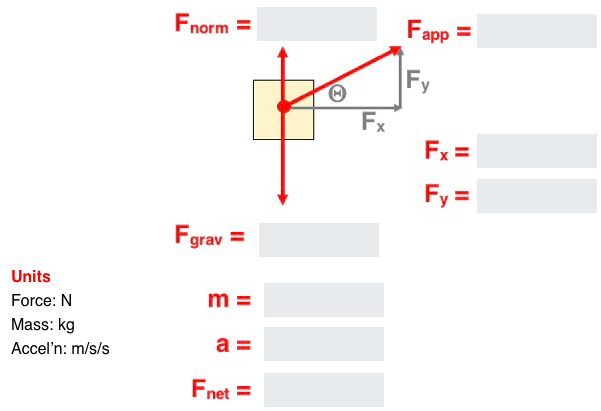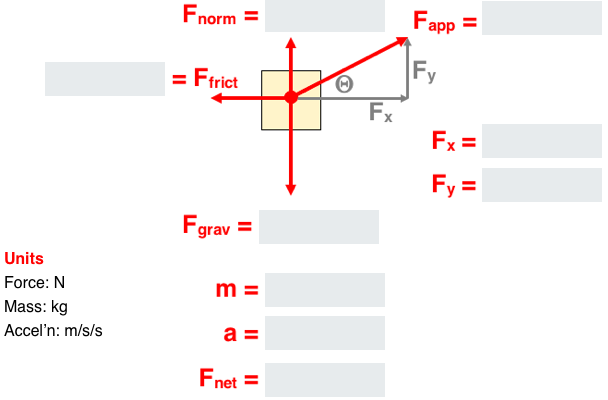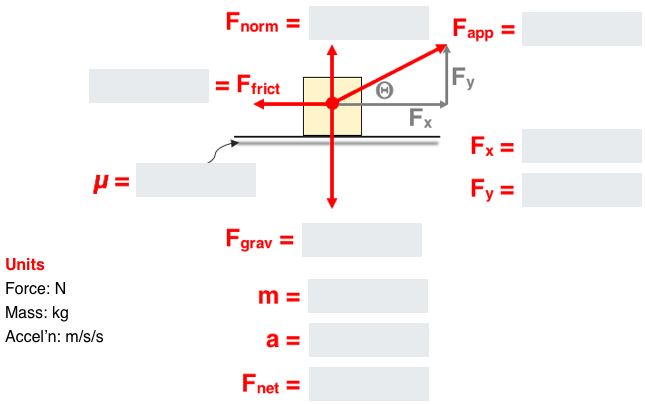## Solve It with Fnet = m•a and Vectors

The Solve It with Fnet = m•a and Vectors Concept Builder includes three groups of two questions spread aross three difficulty levels. Each question requires the analysis of an object being accelerated horizontally by a force exerted at an angle to the horizontal. The analysis involves the use of vector resolution (to determine the components of the angled force) and Newton's second law equation.

The three difficulty levels are differentiated as follows:

• Apprentice Difficulty Level Questions 1-2: Analyze a problem involving a force applied at an angle to the horizontal to cause a horizontal acceleration. No friction.
• Master DifficultyLevel Questions 3-4: Analyze a problem involving a force applied at an angle to the horizontal to cause a horizontal acceleration. Friction value is given.
• Wizard Difficulty Level Questions 5-6: Analyze a problem involving a force applied at an angle to the horizontal to cause a horizontal acceleration. Coefficient of friction is given.

The Physics Classroom grants teachers and other users the right to print these questions for private use. Users are also granted the right to copy the text and modify it for their own use. However, this document nor its graphics should not be uploaded to other servers for distribution to and/or display by others. The Physics Classroom website should remain the only website or server from which the document  and its graphics is distributed or displayed. We also provide a PDF that teachers can use under the same conditions. We have included a link to the PDF near the bottom of this page.

#### Solve It! (with Fnet = m•a and Vectors)

NOTE: Numerical values used in this Concept Builder are randomly generated and likely different than those published here.

Apprentice Difficulty Level
Question 1
Analyze this: A 58.2-N force is applied at an angle of 23.8° above the horizontal to accelerate a 3.15-kg object across a level, friction-free surface. Complete the diagram.Question 2
Analyze this:  A 143-N force is applied at an angle of 39.5° above the horizontal to accelerate a 24.6-kg object across a level, friction-free surface. Complete the diagram.Master Difficulty Level
Question 3
Analyze this:  A 80.4-N force is applied at an angle of 18.8° above the horizontal to accelerate a 5.64-kg object across a level surface. The object encounters 11.2 N of friction. Complete the diagram.Question 4
Analyze this:  A 139-N force is applied at an angle of 26.9° above the horizontal to accelerate a 17.1-kg object across a level surface. The object encounters 31.5 N of friction. Complete the diagram.Wizard Difficulty Level
Question 5
Analyze this:  A 184-N force is applied at an angle of 20.4° above the horizontal to accelerate a 16.9-kg object across a level surface. The coefficient of friction is 0.156. Complete the diagram.Question 6
Analyze this:  A 275-N force is applied at an angle of 26.2° above the horizontal to accelerate a 27.7-kg object across a level surface. The coefficient of friction is 0.286. Complete the diagram.